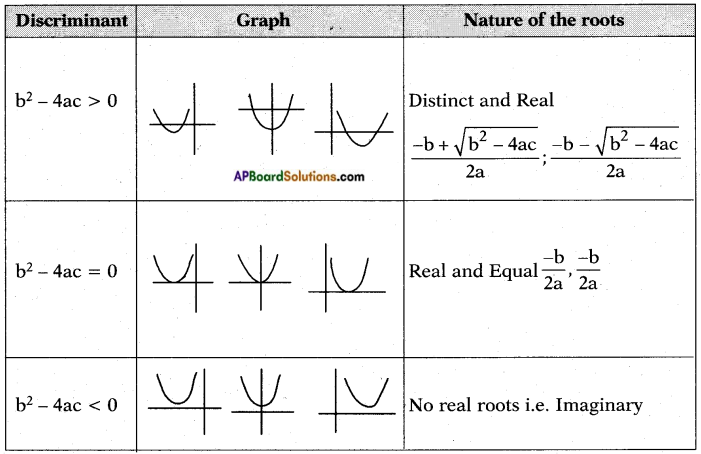Students can go through AP SSC 10th Class Maths Notes Chapter 5 Quadratic Equations to understand and remember the concepts easily.

## AP State Syllabus SSC 10th Class Maths Notes Chapter 5 Quadratic Equations

→ The general form of a linear equation in one variable is ax + b = c.

→ Any equation of the form p(x) = 0 where p(x) is a polynomial of degree 2, is a quadratic equation.→ If p(x) = 0 whose degree is 2 is written in descending order of their degrees, then we say that the quadratic equation is written in the standard form.

→ The standard form of a quadratic equation is ax2 + bx + c = 0 where a ≠ 0. We can write it as y = ax2 + bx + c.

→ There are various occasions in which we make use of Q.E. in our day-to-day life.
Eg : The height of a rocket is defined by a Q.E.

→ Let ax2 + bx + c = 0 be a quadratic equation. A real number α is called a root of the Q.E. if aα2 + bα + c = 0. And x = α is called a solution of the Q.E.
(i.e.) the real value of x for which the Q.E ax2 + bx + c = 0 is satisfied is called its solution.

→ Zeroes of the Q.E. ax2 + bx + c = 0 and the roots of the Q.E. ax2 + bx + c = 0 are the same.

→ To factorise a Q.E. ax2 + bx + c = 0, we find p, q ∈ R such that p + q = b and pq = ac. This process is called Factorising a Q.E. by splitting its middle term.
Eg : 12x2 + 13x + 3 = 0
here a = 12; b = 13; c = 3
a.c = 12 × 3 = 36 = 4 × 9 and
b = 4 + 9 here p = 9 and q = 4
Now 12x2 + 13x + 3 = 0
⇒ 12x2 + 9x + 4x + 3 = 0
⇒ 3x(4x + 3) + 1 (4x + 3) = 0
⇒ (4x + 3) (3x + 1) = 0
Here 4x + 3 = 0 or 3x + 1 = 0
⇒ 4x = -3 or 3x = -1
⇒ x = $$\frac{-3}{4}$$ or $$\frac{-1}{3}$$
$$\frac{-3}{4}$$ and $$\frac{-1}{3}$$ are called the roots of the Q.E. 12x2 + 13x + 3 = 0 and x = $$\frac{-3}{4}$$ or $$\frac{-1}{3}$$ is the solution of the Q.E. 12x2 + 13x + 3 = 0.→ In the above example, (4x + 3) and (3x +1) are called the linear factors of the Q.E. 12x2 + 13x + 3 = 0.

→ We can factorise a Q.E. by adjusting its left side so that it becomes a perfect square.
Eg: x2 + 6x + 8 = 0
⇒ x2 + 2.x.3 + 8 = 0
⇒ x2 + 2.x.3 = -8
The L.H.S. is of the form a2 + 2ab
∴ By adding b2 it becomes a perfect square
∴ x2 + 2.x.3 + 32 = -8 + 32
⇒ (x + 3)2 = -8 + 9
⇒ (x + 3)2 = 1
⇒ x + 3 = ± 1 Now we take x + 3 = 1 or x + 3 = -l
⇒ x = -2 or x = -4

→ Adjusting a Q.E. of the form ax2 + bx + c = 0 so that it becomes a perfect square.
Step – 1 : ax2 + bx + c = 0 ⇒ ax2 + bx = -c ⇒ x2 + $$\frac{b}{a}$$x = $$\frac{-c}{a}$$
Step – 2 :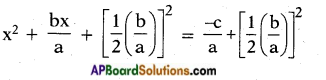Step – 3 :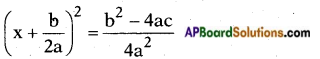Step – 4 : Solve the above.
E.g: 5x2 – 6x + 2 = 0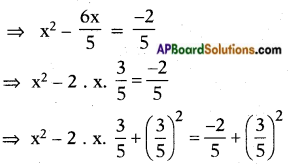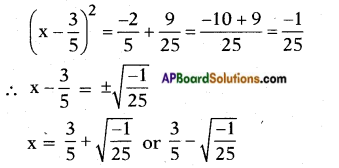→ Let ax2 + bx + c = 0 (a ≠ 0) be a Q.E., then b2 – 4ac is called the Discriminant of the Q.E.

→ If b2 – 4ac > 0, then the roots of the Q.E. ax2 + bx + c = 0 are given by
x = $$\frac{-b \pm \sqrt{b^{2}-4 a c}}{2 a}$$. This is called quadratic formula to find the roots.

The nature of the roots of a Q.E. can be determined either by its discriminant or its graph.
Q.E.: y = ax2 + bx + c.